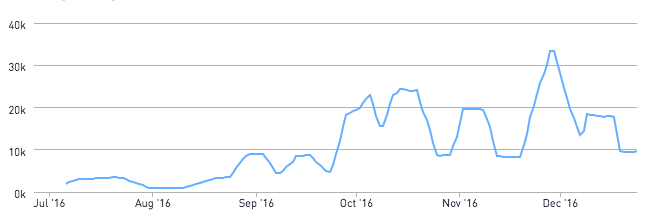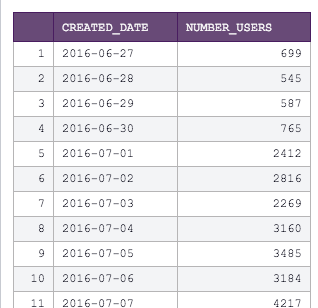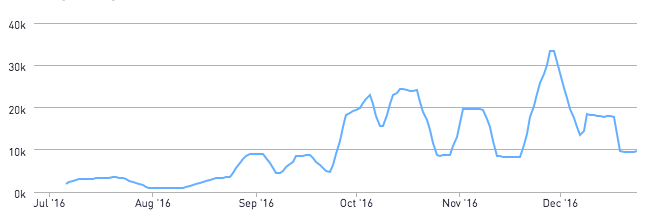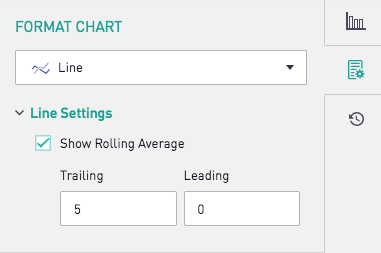# Using R to Plot only the Rolling Average LineWant to show a rolling average to your data? Using the Periscope Data Python / R integration, we can use a few quick lines in R to accomplish this.

Here are the first few rows of our SQL output - a list of dates with the number of users created on a fictional gaming platform.We now use the rollmean() function from the zoo library in R, as shown below. Note from the rollmean() documentation that you can just as easily calculate a host of other rolling calculations, such as a rolling median or sum.

```library(zoo)

# Calculating the rolling average. Window is set to 3 here, corresponding to an average of the current row and the 2 preceding rows
window <- 10
padding <- rep(NA, window - 1)
df\$rollingavg <- c(padding, rollmean(df\$number_users, k = window))

periscope.table(df)```

Now, we make this chart a line graph to visualize the rolling average line by itself!Tip: If you want to display both the raw data and the rolling average, Periscope's built-in visualizations has a quick "Show Rolling Average" check box that you can toggle on.Want to stick to SQL? You can run the same calculation using SQL window functions as shown here

Prefer Python? Find the Python equivalent to this post here.